# GPU Particle Burst#In this example, we show how to create explosions using particles. The particles are tracked by the GPU, significantly improving the performance.

## Step 1: Open a Blank Window#

gpu_particle_burst_01.py#
``` 1"""
2Example showing how to create particle explosions via the GPU.
3"""
5
6SCREEN_WIDTH = 1024
7SCREEN_HEIGHT = 768
8SCREEN_TITLE = "GPU Particle Explosion"
9
10
12    """ Main window"""
13    def __init__(self):
14        super().__init__(SCREEN_WIDTH, SCREEN_HEIGHT, SCREEN_TITLE)
15
16    def on_draw(self):
17        """ Draw everything """
18        self.clear()
19
20    def on_update(self, dt):
21        """ Update everything """
22        pass
23
24    def on_mouse_press(self, x: float, y: float, button: int, modifiers: int):
25        """ User clicks mouse """
26        pass
27
28
29if __name__ == "__main__":
30    window = MyWindow()
31    window.center_window()
```

## Step 2: Create One Particle For Each Click#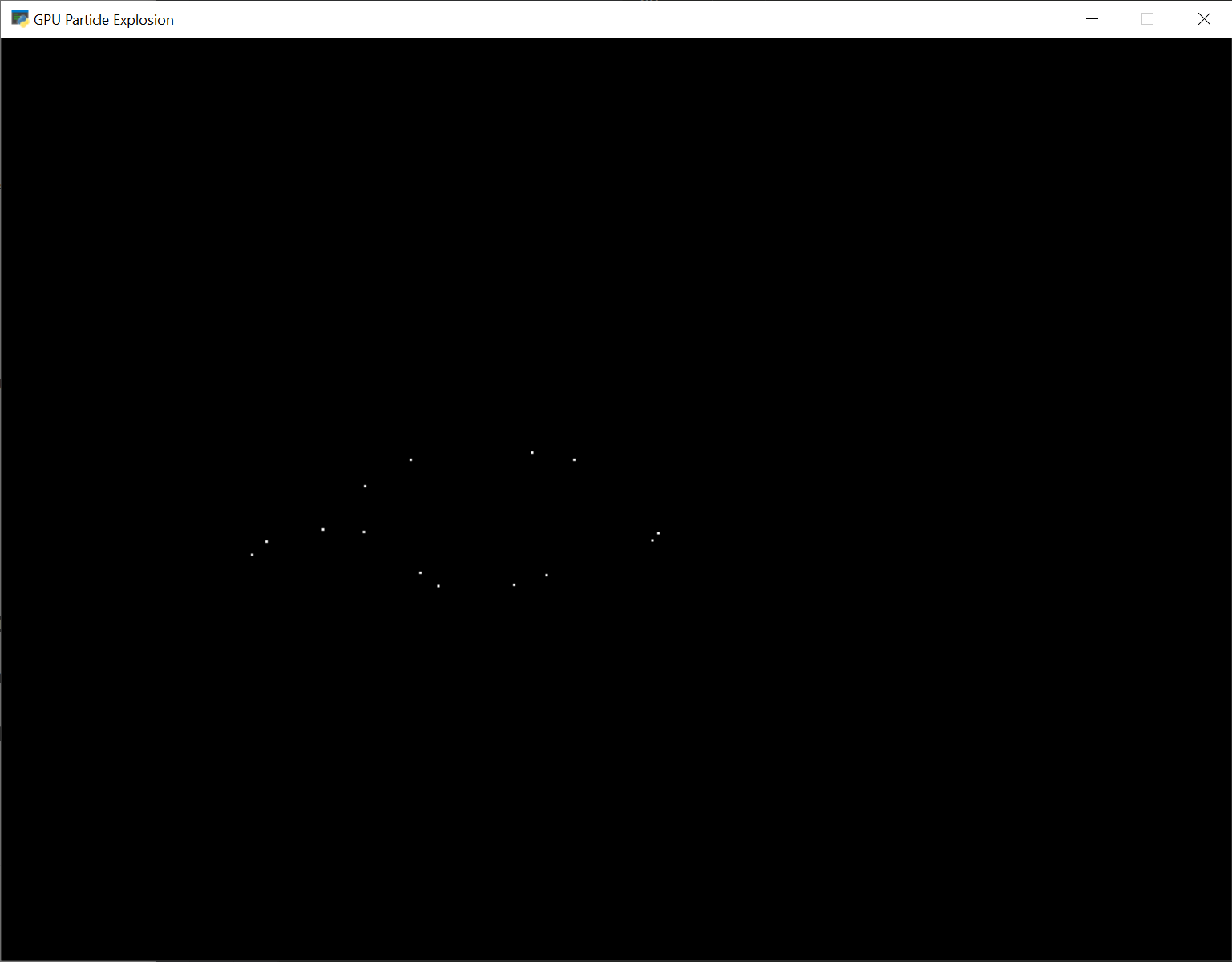For this next section, we are going to draw a dot each time the user clicks their mouse on the screen.

For each click, we are going to create an instance of a `Burst` class that will eventually be turned into a full explosion. Each burst instance will be added to a list.

### Imports#

First, we’ll import some more items for our program:

```from array import array
from dataclasses import dataclass

```

### Burst Dataclass#

Next, we’ll create a dataclass to track our data for each burst. For each burst we need to track a Vertex Array Object (VAO) which stores information about our burst. Inside of that, we’ll have a Vertex Buffer Object (VBO) which will be a high-speed memory buffer where we’ll store locations, colors, velocity, etc.

```@dataclass
class Burst:
""" Track for each burst. """
```

### Init method#

Next, we’ll create an empty list attribute called `burst_list`. We’ll also create our OpenGL shader program. The program will be a collection of two shader programs. These will be stored in separate files, saved in the same directory.

Note

In addition to loading the program via the load_program() method of ArcadeContext shown, it is also possible to keep the GLSL programs in triple- quoted string by using program() of Context.

MyWindow.__init__#
```    def __init__(self):
super().__init__(SCREEN_WIDTH, SCREEN_HEIGHT, SCREEN_TITLE)
self.burst_list = []

# Program to visualize the points
)

self.ctx.enable_only()
```

The OpenGL Shading Language (GLSL) is C-style language that runs on your graphics card (GPU) rather than your CPU. Unfortunately a full explanation of the language is beyond the scope of this tutorial. I hope, however, the tutorial can get you started understanding how it works.

We’ll have two shaders. A vertex shader, and a fragment shader. A vertex shader runs for each vertex point of the geometry we are rendering, and a fragment shader runs for each pixel. For example, vertex shader might run four times for each point on a rectangle, and the fragment shader would run for each pixel on the screen.

The vertex shader takes in the position of our vertex. We’ll set `in_pos` in our Python program, and pass that data to this shader.

The vertex shader outputs the color of our vertex. Colors are in Red-Green-Blue-Alpha (RGBA) format, with floating-point numbers ranging from 0 to 1. In our program below case, we set the color to (1, 1, 1) which is white, and the fourth 1 for completely opaque.

``` 1#version 330
2
3// (x, y) position passed in
4in vec2 in_pos;
5
6// Output the color to the fragment shader
7out vec4 color;
8
9void main() {
10
11    // Set the RGBA color
12    color = vec4(1, 1, 1, 1);
13
14    // Set the position. (x, y, z, w)
15    gl_Position = vec4(in_pos, 0.0, 1);
16}
```

There’s not much to the fragment shader, it just takes in `color` from the vertex shader and passes it back out as the pixel color. We’ll use the same fragment shader for every version in this tutorial.

``` 1#version 330
2
3// Color passed in from the vertex shader
4in vec4 color;
5
6// The pixel we are writing to in the framebuffer
7out vec4 fragColor;
8
9void main() {
10
11    // Fill the point
12    fragColor = vec4(color);
13}
```

### Mouse Pressed#

Each time we press the mouse button, we are going to create a burst at that location.

The data for that burst will be stored in an instance of the `Burst` class.

The `Burst` class needs our data buffer. The data buffer contains information about each particle. In this case, we just have one particle and only need to store the x, y of that particle in the buffer. However, eventually we’ll have hundreds of particles, each with a position, velocity, color, and fade rate. To accommodate creating that data, we have made a generator function `_gen_initial_data`. It is totally overkill at this point, but we’ll add on to it in this tutorial.

The `buffer_description` says that each vertex has two floating data points (`2f`) and those data points will come into the shader with the reference name `in_pos` which we defined above in our OpenGL Shaders

MyWindow.on_mouse_press#
```    def on_mouse_press(self, x: float, y: float, button: int, modifiers: int):
""" User clicks mouse """

def _gen_initial_data(initial_x, initial_y):
""" Generate data for each particle """
yield initial_x
yield initial_y

# Recalculate the coordinates from pixels to the OpenGL system with
# 0, 0 at the center.
x2 = x / self.width * 2. - 1.
y2 = y / self.height * 2. - 1.

# Get initial particle data
initial_data = _gen_initial_data(x2, y2)

# Create a buffer with that data
buffer = self.ctx.buffer(data=array('f', initial_data))

# Create a buffer description specifying the buffer's data format
buffer,
'2f',
['in_pos'])

# Create our Vertex Attribute Object
vao = self.ctx.geometry([buffer_description])

# Create the Burst object and add it to the list of bursts
burst = Burst(buffer=buffer, vao=vao)
self.burst_list.append(burst)
```

### Drawing#

Finally, draw it.

MyWindow.on_draw#
```    def on_draw(self):
""" Draw everything """
self.clear()

# Set the particle size
self.ctx.point_size = 2 * self.get_pixel_ratio()

# Loop through each burst
for burst in self.burst_list:

# Render the burst
burst.vao.render(self.program, mode=self.ctx.POINTS)
```

## Step 3: Multiple Moving Particles#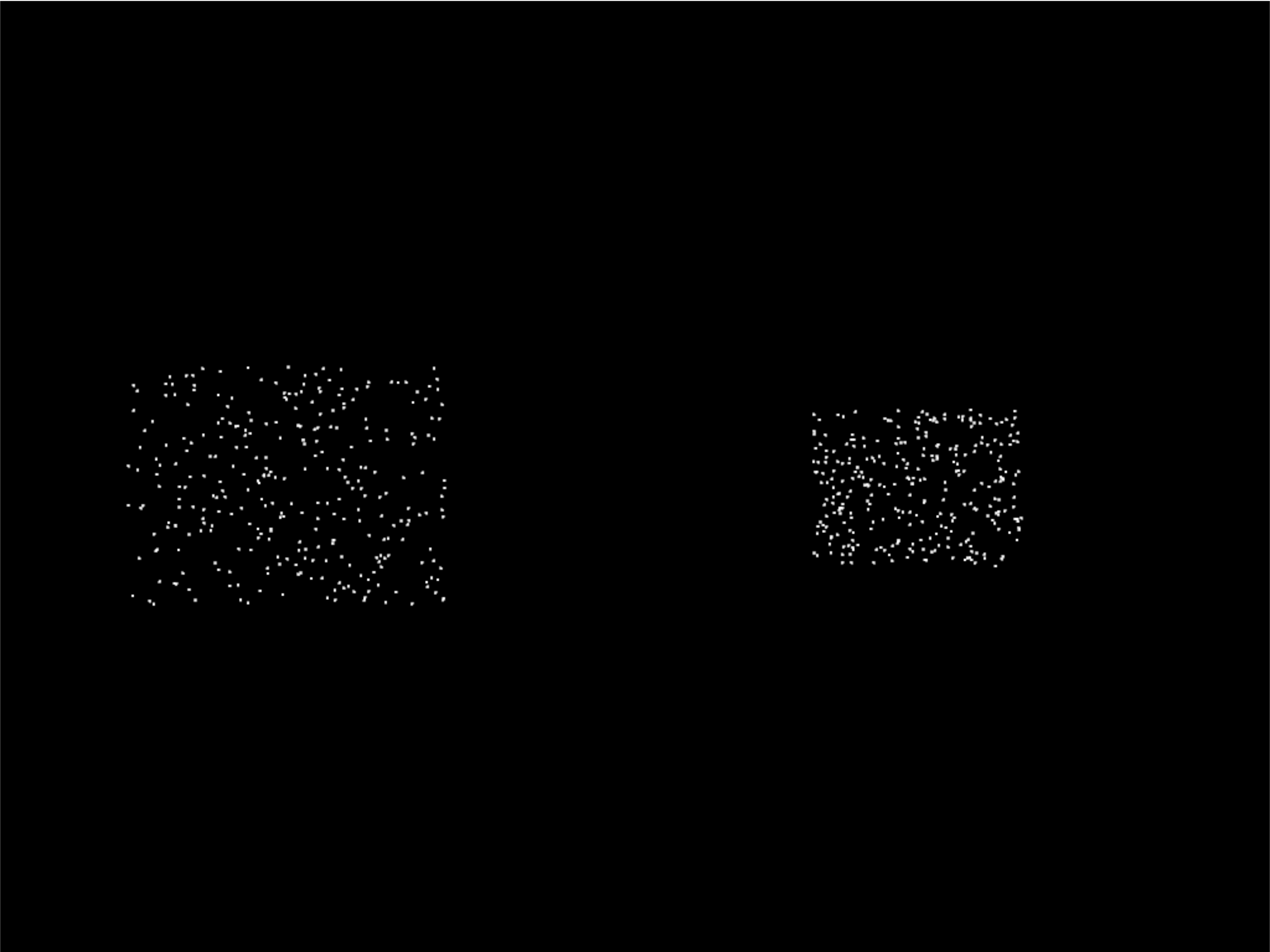Next step is to have more than one particle, and to have the particles move. We’ll do this by creating the particles, and calculating where they should be based on the time since creation. This is a bit different than the way we move sprites, as they are manually repositioned bit-by-bit during each update call.

### Imports#

First, we’ll add imports for both the `random` and `time` libraries:

```import random
import time
```

### Constants#

Then we need to create a constant that contains the number of particles to create:

```PARTICLE_COUNT = 300
```

### Burst Dataclass#

We’ll need to add a time to our burst data. This will be a floating point number that represents the start-time of when the burst was created.

```@dataclass
class Burst:
""" Track for each burst. """
start_time: float
```

### Update Burst Creation#

Now when we create a burst, we need multiple particles, and each particle also needs a velocity. In `_gen_initial_data` we add a loop for each particle, and also output a delta x and y.

Note: Because of how we set delta x and delta y, the particles will expand into a rectangle rather than a circle. We’ll fix that on a later step.

Because we added a velocity, our buffer now needs two pairs of floats `2f 2f` named `in_pos` and `in_vel`. We’ll update our shader in a bit to work with the new values.

Finally, our burst object needs to track the time we created the burst.

``` 1    def on_mouse_press(self, x: float, y: float, button: int, modifiers: int):
2        """ User clicks mouse """
3
4        def _gen_initial_data(initial_x, initial_y):
5            """ Generate data for each particle """
6            for i in range(PARTICLE_COUNT):
7                dx = random.uniform(-.2, .2)
8                dy = random.uniform(-.2, .2)
9                yield initial_x
10                yield initial_y
11                yield dx
12                yield dy
13
14        # Recalculate the coordinates from pixels to the OpenGL system with
15        # 0, 0 at the center.
16        x2 = x / self.width * 2. - 1.
17        y2 = y / self.height * 2. - 1.
18
19        # Get initial particle data
20        initial_data = _gen_initial_data(x2, y2)
21
22        # Create a buffer with that data
23        buffer = self.ctx.buffer(data=array('f', initial_data))
24
25        # Create a buffer description specifying the buffer's data format
27            buffer,
28            '2f 2f',
29            ['in_pos', 'in_vel'])
30
31        # Create our Vertex Attribute Object
32        vao = self.ctx.geometry([buffer_description])
33
34        # Create the Burst object and add it to the list of bursts
35        burst = Burst(buffer=buffer, vao=vao, start_time=time.time())
36        self.burst_list.append(burst)
```

### Set Time in on_draw#

When we draw, we need to set “uniform data” (data that is the same for all points) that says how many seconds it has been since the burst started. The shader will use this to calculate particle position.

```    def on_draw(self):
""" Draw everything """
self.clear()

# Set the particle size
self.ctx.point_size = 2 * self.get_pixel_ratio()

# Loop through each burst
for burst in self.burst_list:

# Set the uniform data
self.program['time'] = time.time() - burst.start_time

# Render the burst
burst.vao.render(self.program, mode=self.ctx.POINTS)
```

Our vertex shader needs to be updated. We now take in a `uniform float` called time. Uniform data is set once, and each vertex in the program can use it. In our case, we don’t need a separate copy of the burst’s start time for each particle in the burst, therefore it is uniform data.

We also need to add another vector of two floats that will take in our velocity. We set `in_vel` in Update Burst Creation.

Then finally we calculate a new position based on the time and our particle’s velocity. We use that new position when setting `gl_Position`.

``` 1#version 330
2
3// Time since burst start
4uniform float time;
5
6// (x, y) position passed in
7in vec2 in_pos;
8
9// Velocity of particle
10in vec2 in_vel;
11
12// Output the color to the fragment shader
13out vec4 color;
14
15void main() {
16
17    // Set the RGBA color
18    color = vec4(1, 1, 1, 1);
19
20    // Calculate a new position
21    vec2 new_pos = in_pos + (time * in_vel);
22
23    // Set the position. (x, y, z, w)
24    gl_Position = vec4(new_pos, 0.0, 1);
25}
```

## Step 4: Random Angle and Speed#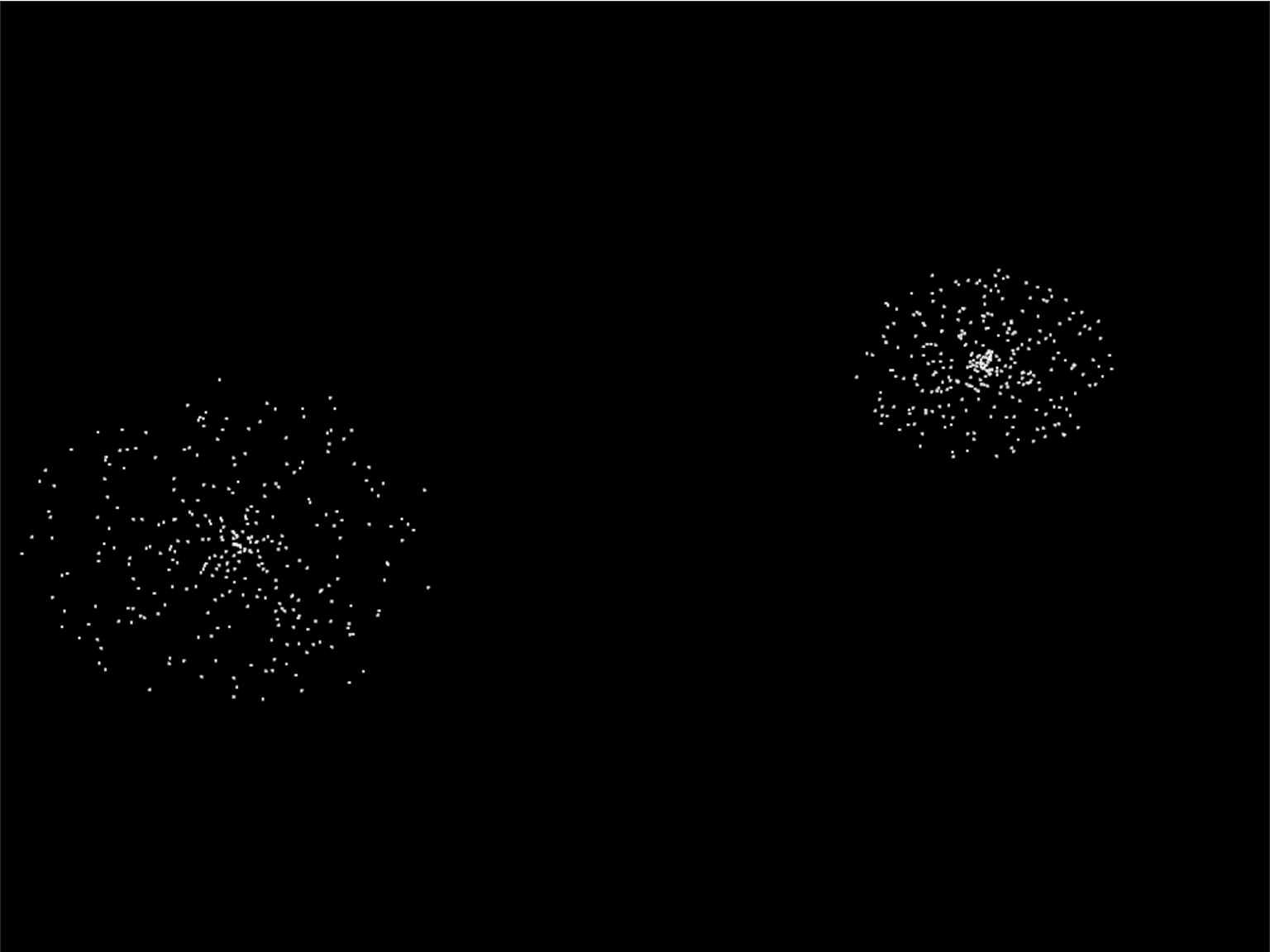Step 3 didn’t do a good job of picking a velocity, as our particles expanded into a rectangle rather than a circle. Rather than just pick a random delta x and y, we need to pick a random direction and speed. Then calculate delta x and y from that.

### Update Imports#

Import the math library so we can do some trig:

```import math
```

### Update Burst Creation#

Now, pick a random direction from zero to 2 pi radians. Also, pick a random speed. Then use sine and cosine to calculate the delta x and y.

``` 1    def on_mouse_press(self, x: float, y: float, button: int, modifiers: int):
2        """ User clicks mouse """
3
4        def _gen_initial_data(initial_x, initial_y):
5            """ Generate data for each particle """
6            for i in range(PARTICLE_COUNT):
7                angle = random.uniform(0, 2 * math.pi)
8                speed = random.uniform(0.0, 0.3)
9                dx = math.sin(angle) * speed
10                dy = math.cos(angle) * speed
11                yield initial_x
12                yield initial_y
13                yield dx
14                yield dy
15
```

## Step 5: Gaussian Distribution#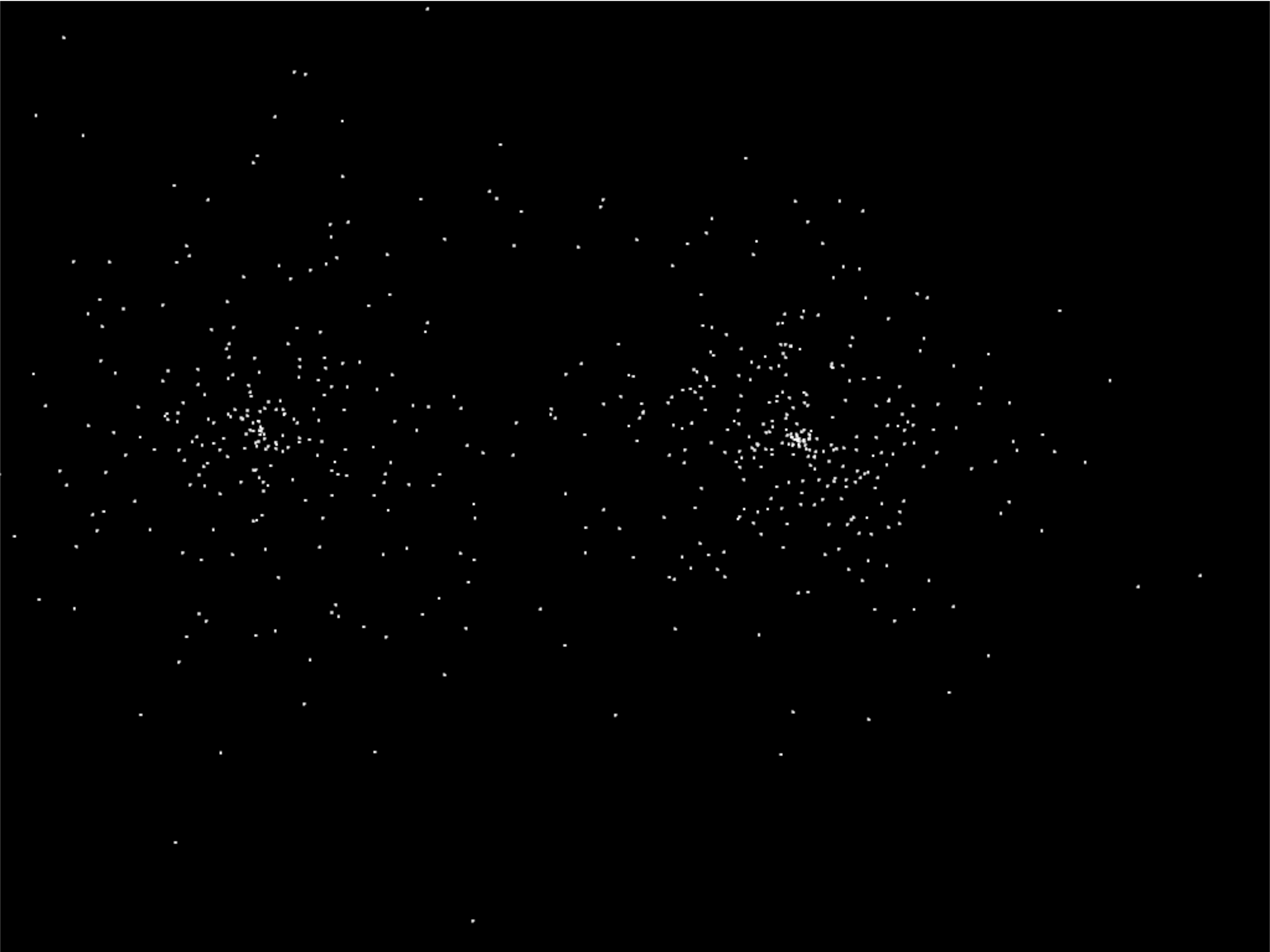Setting speed to a random amount makes for an expanding circle. Another option is to use a gaussian function to produce more of a ‘splat’ look:

```                speed = abs(random.gauss(0, 1)) * .5
```

### Program Listings#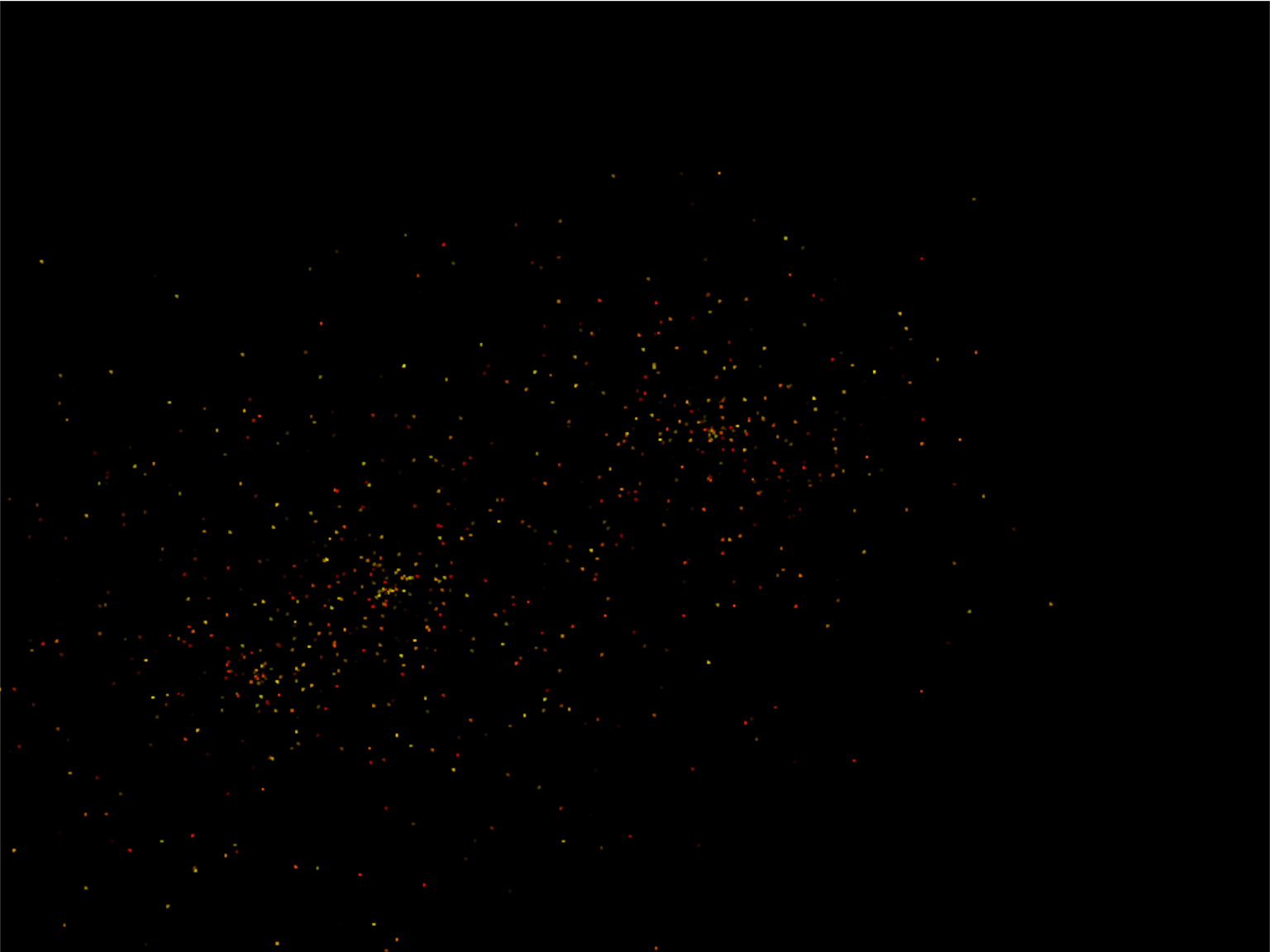So far our particles have all been white. How do we add in color? We’ll need to generate it for each particle. Shaders take colors in the form of RGB floats, so we’ll generate a random number for red, and add in some green to get our yellows. Don’t add more green than red, or else you get a green tint.

Finally, make sure to update the shader buffer description (VBO) to accept the three color channel floats (`3f`) under the name `in_color`.

``` 1    def on_mouse_press(self, x: float, y: float, button: int, modifiers: int):
2        """ User clicks mouse """
3
4        def _gen_initial_data(initial_x, initial_y):
5            """ Generate data for each particle """
6            for i in range(PARTICLE_COUNT):
7                angle = random.uniform(0, 2 * math.pi)
8                speed = abs(random.gauss(0, 1)) * .5
9                dx = math.sin(angle) * speed
10                dy = math.cos(angle) * speed
11                red = random.uniform(0.5, 1.0)
12                green = random.uniform(0, red)
13                blue = 0
14                yield initial_x
15                yield initial_y
16                yield dx
17                yield dy
18                yield red
19                yield green
20                yield blue
21
22        # Recalculate the coordinates from pixels to the OpenGL system with
23        # 0, 0 at the center.
24        x2 = x / self.width * 2. - 1.
25        y2 = y / self.height * 2. - 1.
26
27        # Get initial particle data
28        initial_data = _gen_initial_data(x2, y2)
29
30        # Create a buffer with that data
31        buffer = self.ctx.buffer(data=array('f', initial_data))
32
33        # Create a buffer description specifying the buffer's data format
35            buffer,
36            '2f 2f 3f',
37            ['in_pos', 'in_vel', 'in_color'])
38
39        # Create our Vertex Attribute Object
40        vao = self.ctx.geometry([buffer_description])
41
42        # Create the Burst object and add it to the list of bursts
43        burst = Burst(buffer=buffer, vao=vao, start_time=time.time())
44        self.burst_list.append(burst)
```

Then, update the shader to use the color instead of always using white:

``` 1#version 330
2
3// Time since burst start
4uniform float time;
5
6// (x, y) position passed in
7in vec2 in_pos;
8
9// Velocity of particle
10in vec2 in_vel;
11
12// Color of particle
13in vec3 in_color;
14
15// Output the color to the fragment shader
16out vec4 color;
17
18void main() {
19
20    // Set the RGBA color
21    color = vec4(in_color, in_color, in_color, 1);
22
23    // Calculate a new position
24    vec2 new_pos = in_pos + (time * in_vel);
25
26    // Set the position. (x, y, z, w)
27    gl_Position = vec4(new_pos, 0.0, 1);
28}
```

### Program Listings#Right now the explosion particles last forever. Let’s get them to fade out. Once a burst has faded out, let’s remove it from `burst_list`.

### Constants#

First, let’s add a couple constants to control the minimum and maximum times to fade a particle:

```MIN_FADE_TIME = 0.25
```

### Update Init#

Next, we need to update our OpenGL context to support alpha blending. Go back to the `__init__` method and update the `enable_only` call to:

```self.ctx.enable_only(self.ctx.BLEND)
```

``` 1    def on_mouse_press(self, x: float, y: float, button: int, modifiers: int):
2        """ User clicks mouse """
3
4        def _gen_initial_data(initial_x, initial_y):
5            """ Generate data for each particle """
6            for i in range(PARTICLE_COUNT):
7                angle = random.uniform(0, 2 * math.pi)
8                speed = abs(random.gauss(0, 1)) * .5
9                dx = math.sin(angle) * speed
10                dy = math.cos(angle) * speed
11                red = random.uniform(0.5, 1.0)
12                green = random.uniform(0, red)
13                blue = 0
16
17                yield initial_x
18                yield initial_y
19                yield dx
20                yield dy
21                yield red
22                yield green
23                yield blue
25
26        # Recalculate the coordinates from pixels to the OpenGL system with
27        # 0, 0 at the center.
28        x2 = x / self.width * 2. - 1.
29        y2 = y / self.height * 2. - 1.
30
31        # Get initial particle data
32        initial_data = _gen_initial_data(x2, y2)
33
34        # Create a buffer with that data
35        buffer = self.ctx.buffer(data=array('f', initial_data))
36
37        # Create a buffer description specifying the buffer's data format
39            buffer,
40            '2f 2f 3f f',
42
43        # Create our Vertex Attribute Object
44        vao = self.ctx.geometry([buffer_description])
45
46        # Create the Burst object and add it to the list of bursts
47        burst = Burst(buffer=buffer, vao=vao, start_time=time.time())
48        self.burst_list.append(burst)
```

Update the shader. Calculate the alpha. If it is less that 0, just use 0.

``` 1#version 330
2
3// Time since burst start
4uniform float time;
5
6// (x, y) position passed in
7in vec2 in_pos;
8
9// Velocity of particle
10in vec2 in_vel;
11
12// Color of particle
13in vec3 in_color;
14
17
18// Output the color to the fragment shader
19out vec4 color;
20
21void main() {
22
23    // Calculate alpha based on time and fade rate
24    float alpha = 1.0 - (in_fade_rate * time);
25    if(alpha < 0.0) alpha = 0;
26
27    // Set the RGBA color
28    color = vec4(in_color, in_color, in_color, alpha);
29
30    // Calculate a new position
31    vec2 new_pos = in_pos + (time * in_vel);
32
33    // Set the position. (x, y, z, w)
34    gl_Position = vec4(new_pos, 0.0, 1);
35}
```

Once our burst has completely faded, no need to keep it around. So in our `on_update` remove the burst from the burst_list after it has been faded.

``` 1    def on_update(self, dt):
2        """ Update game """
3
4        # Create a copy of our list, as we can't modify a list while iterating
5        # it. Then see if any of the items have completely faded out and need
6        # to be removed.
7        temp_list = self.burst_list.copy()
8        for burst in temp_list:
9            if time.time() - burst.start_time > MAX_FADE_TIME:
10                self.burst_list.remove(burst)
```

### Program Listings#

```// Adjust velocity based on gravity